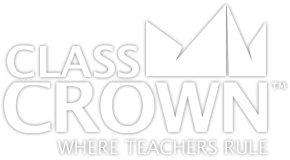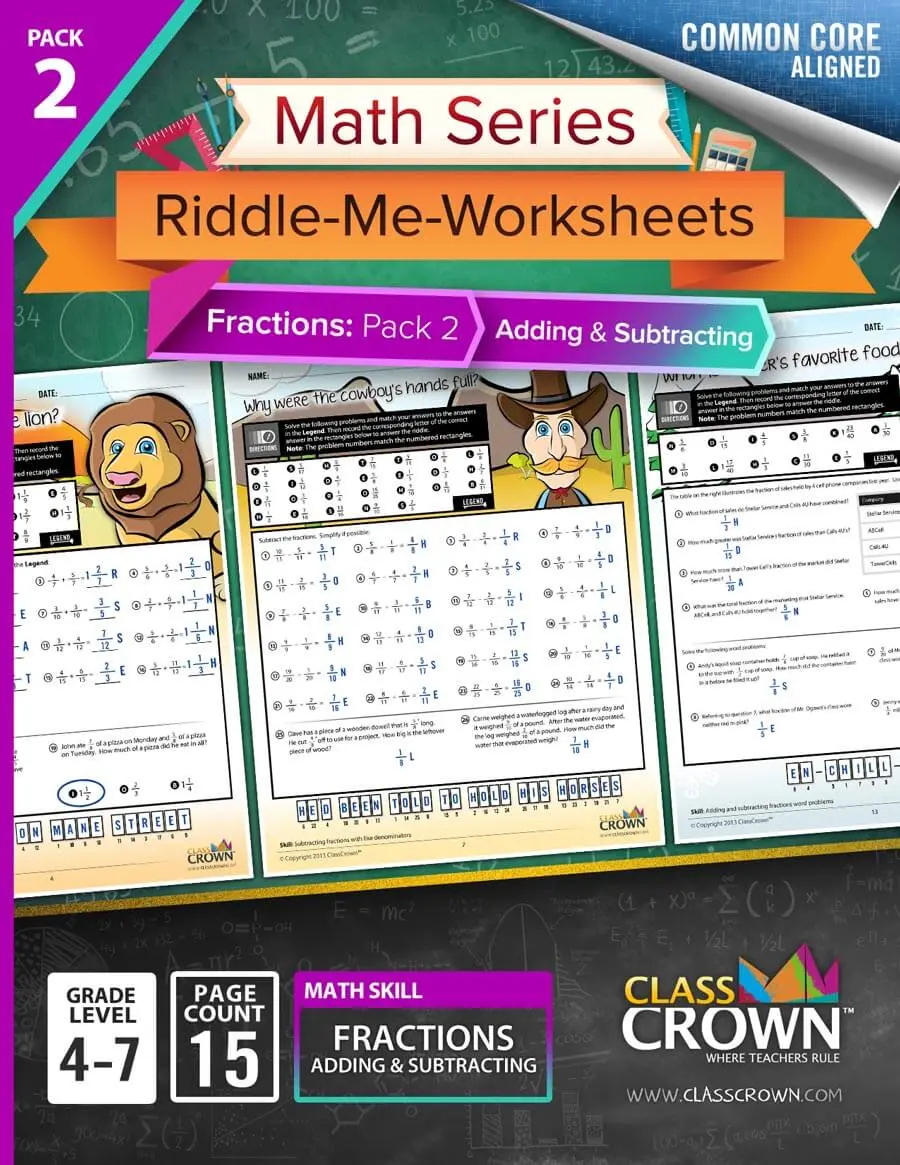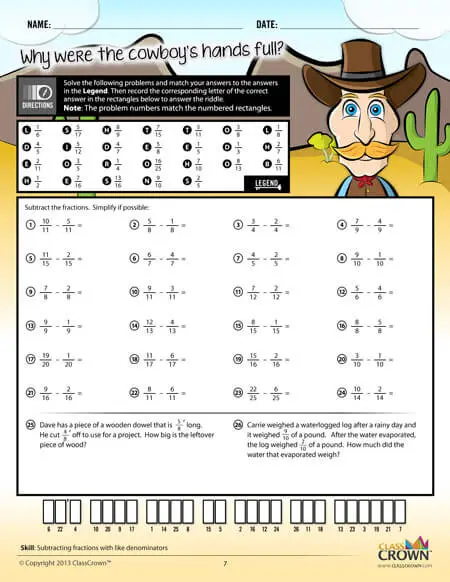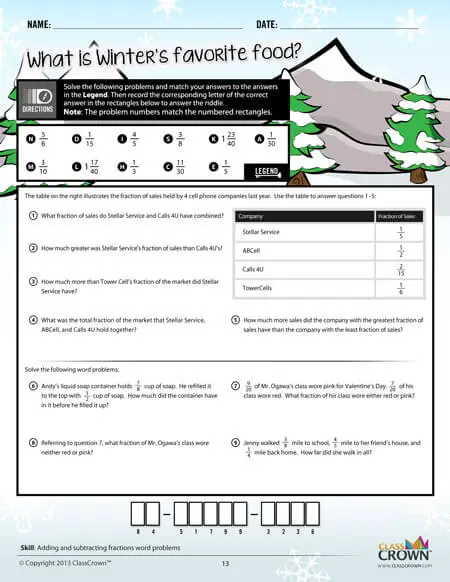Math Worksheets

# Fractions Pack 2: Adding & Subtracting

## Self-checking math worksheets with riddles to keep kids motivated.

Rating: 5/5
(0 Reviews)
4th – 7th
WORKSHEET COUNT
15
FORMAT(S)
PDF
Yes
\$5.99
Decimals & Fractions Bundle Pack
This bundle includes decimals pack 1, and fractions packs 1-3.
\$17.99
\$23.96
Save 24%
The Details
Description
• Do your students ever complete an entire math worksheet, only to find that they have done most of the problems incorrectly and clearly have a misconception?
• ClassCrown’s Riddle-Me-Worksheets help to prevent this problem by introducing a puzzle aspect to math, giving students immediate feedback as to whether or not they are solving problems correctly. If the answer to the riddle isn't correct, the student knows which problems he's made an error on.
Specifics
• Subject:  Fractions
• Product Count:  15
• Full Page Count:  26
• File Format(s):  PDF

• Grade Levle(s):  4th, 5th, 6th, 7th
What's Included?
• Adding fractions with like denominators
• Adding mixed numbers with like denominators, no regrouping
• Adding fractions with unlike denominators
• Subtracting fractions with like denominators
• Subtracting mixed numbers with like denominators
• Subtracting fractions with unlike denominators
• Adding mixed numbers with unlike denominators
• Subtracting mixed numbers from whole numbers
• Subtracting fractions with unlike denominators and with regrouping
• Adding and subtracting fractions word problems
• Adding and subtracting mixed numbers word problems
• Adding/subtracting fractions and mixed numbers
• Adding three or more fractions with unlike denominators
• Inequalities with adding and subtracting fractions
• Estimating sums of fractions
Reviews
Overall Rating: 5/5
(Showing 0 of 0)
See More Reviews @ TpTSelf Checking

Our math worksheets introduce a puzzle aspect to math, giving students immediate feedback as to whether or not they are solving problems correctly. If the answer to the riddle isn't spelled correctly, the student knows which problems he's made an error on.Fun Puzzle AspectImmediate Feedback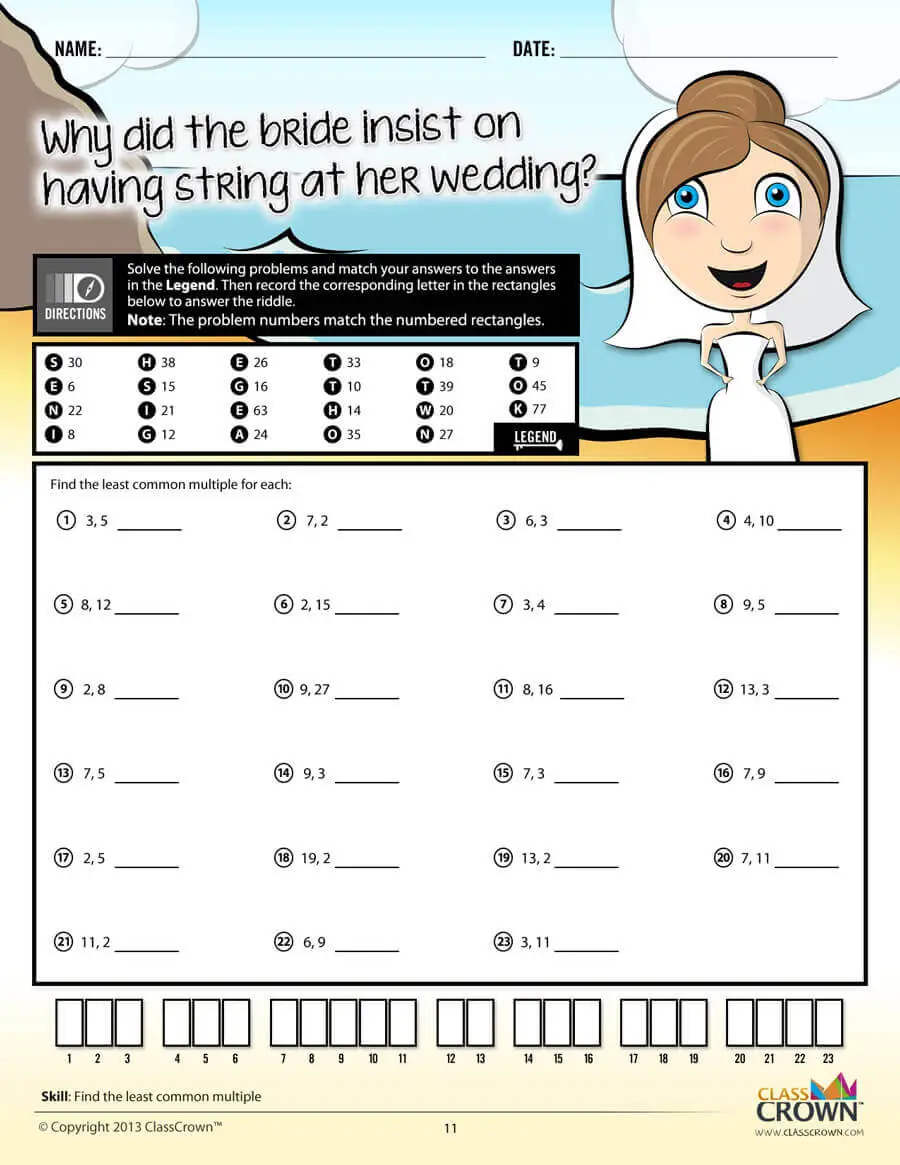Problem Solving Motivation

Each math worksheet contains a riddle that the student solves by completeing all the problems on the worksheet. This keeps kids motivated to complete each problem so that they can find the answer to the riddle.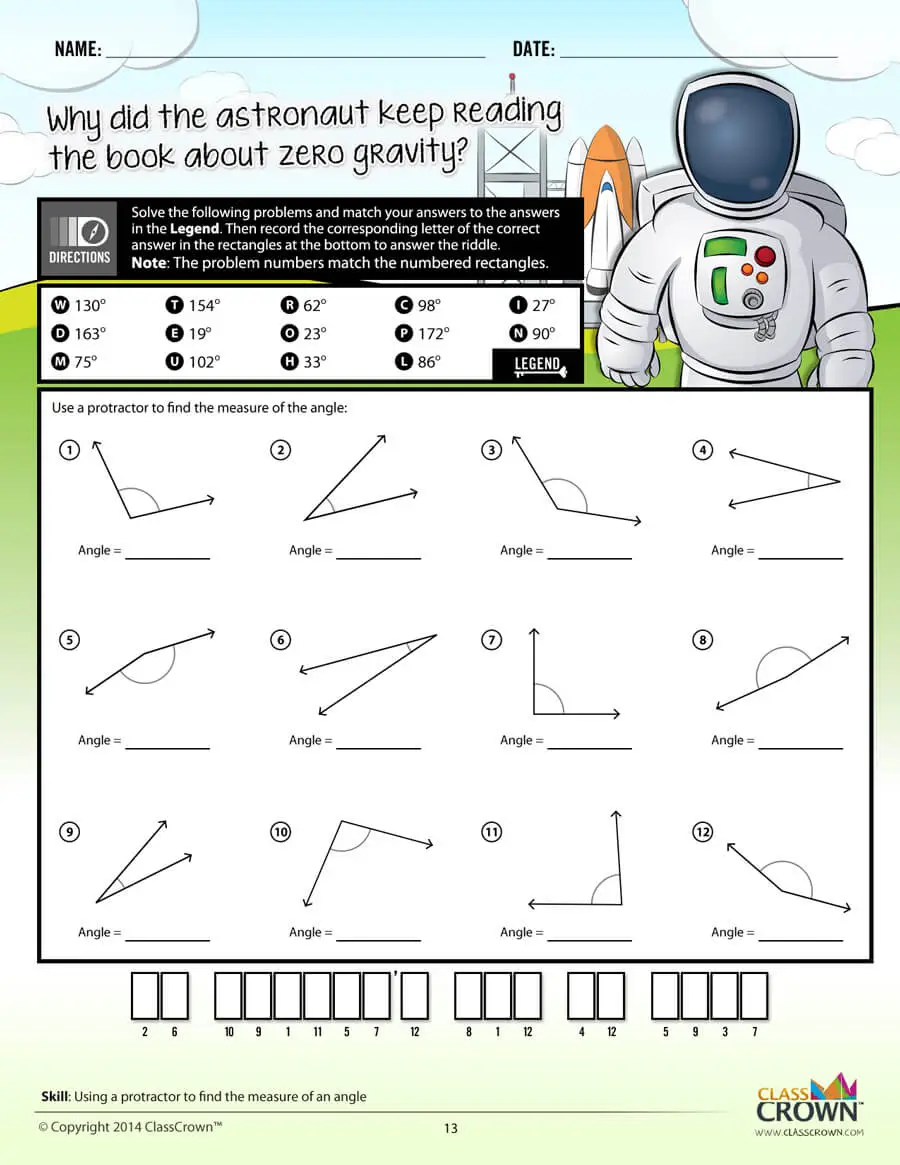Common Core Aligned

All our math worksheet packs are designed with Common Core in mind. That way you don’t have to worry about whether your math ciriculum is aligned or not when you incorpoate ClassCrown Riddle-Me-Worksheets in your lesson plans.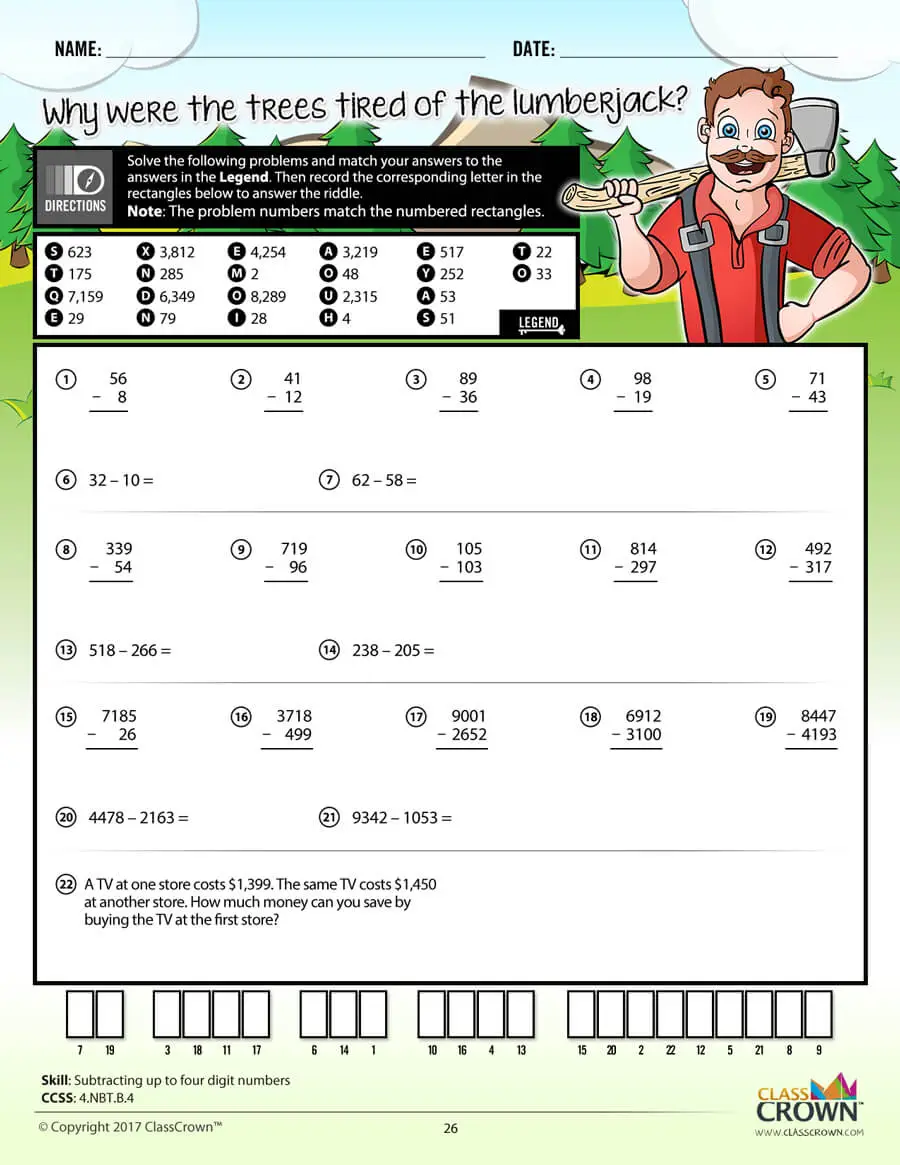High Quality Design

Each page of our math worksheets has been produced in high resolution at 144 dpi and designed in full, vibrant color for maximum quality. They look stunning whether you are printing in color or black and white.High Resolution
(144 dpi)Stunning Color & Clarity